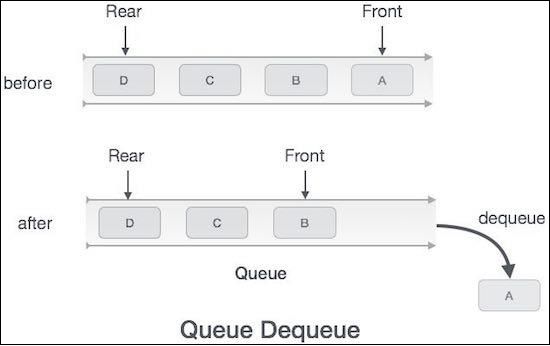# Remove elements from a queue using Javascript

Dequeuing elements from a Queue means removing them from the front/head of the queue. We are taking the start of the container array to be the head of the queue as we'll perform all operations with respect to it.Hence, we can implement the pop function as follows −

## Example

dequeue() {
// Check if empty
if (this.isEmpty()) {
console.log("Queue Underflow!");
return;
}
return this.container.shift();
}

You can check if this function is working fine using −

## Example

let q = new Queue(2);
q.dequeue();
q.enqueue(3);
q.enqueue(4);
console.log(q.dequeue());
q.display();

## Output

This will give the output −

Queue Underflow!
3
[ 4 ]

As you can see from here, 3 went into the queue first, then 4 went in. When we dequeued it, 3 was removed. If this seems less intuitive to you, you can also make the insertions at the beginning and deletions at the end. We'll continue using this convention.